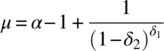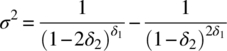# 31Gamma Distribution

As a commonly used distribution, we covered the gamma distribution in detail in Section 12.10. This chapter describes the range of possible modifications.

## 31.1 Inverse Gamma

The inverse gamma distribution is also known as the Pearson‐V distribution and was covered in Section 27.5.

## 31.2 Log‐Gamma

There are at least five distributions that are described as the log‐gamma distribution. In no particular order, the PDF of the first is

(31.1)Raw moments are given by

(31.2)(31.3)(31.4)By replacing δ2 with 1/δ2, we obtain the same distribution that might, at first glance, appear different

(31.5)Figure 31.1 plots Equation (31.5) and shows, with α fixed at zero, the effect of varying δ1 and δ2.Figure 31.1 Log‐gamma (1): Effect of δ1 and δ2 on shape

Raw moments are given by

(31.6)